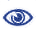# Prague Stringology Conference 2014

Closed Factorization

 Abstract: A closed string is a string with a proper substring that occurs in the string as a prefix and a suffix, but not elsewhere. Closed strings were introduced by Fici (Proc. WORDS, 2011) as objects of combinatorial interest in the study of Trapezoidal and Sturmian words. In this paper we consider algorithms for computing closed factors (substrings) in strings, and in particular for greedily factorizing a string into a sequence of longest closed factors. We describe an algorithm for this problem that uses linear time and space. We then consider the related problem of computing, for every position in the string, the longest closed factor starting at that position. We describe a simple algorithm for the problem that runs in O(n log n / log log n) time.

 Download paper:PostScript PDF BibTeX reference
 Download presentation: